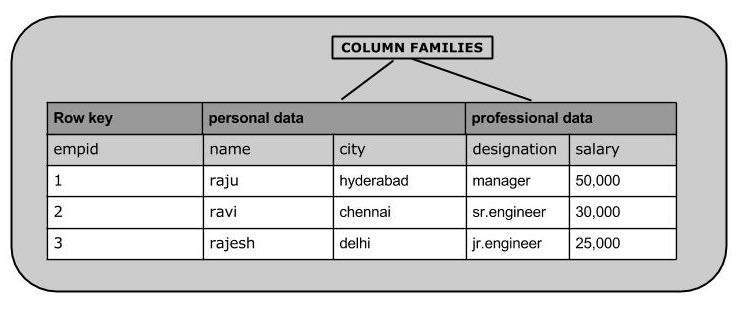# HBase - Create Data

## Inserting Data using HBase Shell

This chapter demonstrates how to create data in an HBase table. To create data in an HBase table, the following commands and methods are used:

• put command,

• add() method of Put class, and

• put() method of HTable class.

As an example, we are going to create the following table in HBase.Using put command, you can insert rows into a table. Its syntax is as follows:

```put ’<table name>’,’row1’,’<colfamily:colname>’,’<value>’
```

### Inserting the First Row

Let us insert the first row values into the emp table as shown below.

```hbase(main):005:0> put 'emp','1','personal data:name','raju'
0 row(s) in 0.6600 seconds
0 row(s) in 0.0410 seconds
hbase(main):007:0> put 'emp','1','professional
data:designation','manager'
0 row(s) in 0.0240 seconds
hbase(main):007:0> put 'emp','1','professional data:salary','50000'
0 row(s) in 0.0240 seconds
```

Insert the remaining rows using the put command in the same way. If you insert the whole table, you will get the following output.

```hbase(main):022:0> scan 'emp'

ROW                        COLUMN+CELL

1 column=personal data:name, timestamp=1417524185058, value=ramu

1 column=professional data:designation, timestamp=1417524232601,

value=manager

1 column=professional data:salary, timestamp=1417524244109, value=50000

2 column=personal data:city, timestamp=1417524574905, value=chennai

2 column=personal data:name, timestamp=1417524556125, value=ravi

2 column=professional data:designation, timestamp=1417524592204,

value=sr:engg

2 column=professional data:salary, timestamp=1417524604221, value=30000

3 column=personal data:city, timestamp=1417524681780, value=delhi

3 column=personal data:name, timestamp=1417524672067, value=rajesh

3 column=professional data:designation, timestamp=1417524693187,

value=jr:engg
3 column=professional data:salary, timestamp=1417524702514,

value=25000
```

## Inserting Data Using Java API

You can insert data into Hbase using the add() method of the Put class. You can save it using the put() method of the HTable class. These classes belong to the org.apache.hadoop.hbase.client package. Below given are the steps to create data in a Table of HBase.

### Step 1:Instantiate the Configuration Class

The Configuration class adds HBase configuration files to its object. You can create a configuration object using the create() method of the HbaseConfiguration class as shown below.

```Configuration conf = HbaseConfiguration.create();
```

### Step 2:Instantiate the HTable Class

You have a class called HTable, an implementation of Table in HBase. This class is used to communicate with a single HBase table. While instantiating this class, it accepts configuration object and table name as parameters. You can instantiate HTable class as shown below.

```HTable hTable = new HTable(conf, tableName);
```

### Step 3: Instantiate the PutClass

To insert data into an HBase table, the add() method and its variants are used. This method belongs to Put, therefore instantiate the put class. This class requires the row name you want to insert the data into, in string format. You can instantiate the Put class as shown below.

```Put p = new Put(Bytes.toBytes("row1"));
```

### Step 4: Insert Data

The add() method of Put class is used to insert data. It requires 3 byte arrays representing column family, column qualifier (column name), and the value to be inserted, respectively. Insert data into the HBase table using the add() method as shown below.

```p.add(Bytes.toBytes("coloumn family "), Bytes.toBytes("column
name"),Bytes.toBytes("value"));
```

### Step 5: Save the Data in Table

After inserting the required rows, save the changes by adding the put instance to the put() method of HTable class as shown below.

```hTable.put(p);
```

### Step 6: Close the HTable Instance

After creating data in the HBase Table, close the HTable instance using the close() method as shown below.

```hTable.close();
```

Given below is the complete program to create data in HBase Table.

```import java.io.IOException;

public class InsertData{

public static void main(String[] args) throws IOException {

// Instantiating Configuration class
Configuration config = HBaseConfiguration.create();

// Instantiating HTable class
HTable hTable = new HTable(config, "emp");

// Instantiating Put class
// accepts a row name.
Put p = new Put(Bytes.toBytes("row1"));

// accepts column family name, qualifier/row name ,value
Bytes.toBytes("name"),Bytes.toBytes("raju"));

Bytes.toBytes("manager"));

Bytes.toBytes("50000"));

// Saving the put Instance to the HTable.
hTable.put(p);
System.out.println("data inserted");

// closing HTable
hTable.close();
}
}
```

Compile and execute the above program as shown below.

```\$javac InsertData.java
\$java InsertData
```

The following should be the output:

```data inserted
```